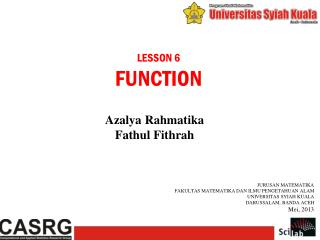DownloadDownload PresentationLESSON 6 FUNCTION

# LESSON 6 FUNCTION

Download Presentation## LESSON 6 FUNCTION

- - - - - - - - - - - - - - - - - - - - - - - - - - - E N D - - - - - - - - - - - - - - - - - - - - - - - - - - -
##### Presentation Transcript

1. LESSON 6 FUNCTION AzalyaRahmatika FathulFithrah JURUSAN MATEMATIKA FAKULTAS MATEMATIKA DAN ILMU PENGETAHUAN ALAM UNIVERSITAS SYIAH KUALA DARUSSALAM, BANDA ACEH Mei, 2013

2. Overview In this section, we present Scilab function. We analyze the way to define a new function and the method to load it into Scilab. Where the following list presents the various variables used in the syntax: • myfunction is the name of the function, • invar is the name of the input arguments, • outvar is the name of the output arguments.

3. The values of the input arguments are not modified by the function, while the values of the output arguments are actually modified by the function. We have in fact already met several functions in this document. The sin function, in the y = sin (x) statement, takes the input argument x and returns the result in the output argument y. in Scilab vocabulary, the input arguments are called the right hand side and the output arguments are called the left hand side.

4. Functions can have an arbitrary number of input and output arguments so that the complete syntax for a function which has a fixed number of arguments is the following: The input and output arguments are separated by commas “,”. Notice that the input arguments are surrounded by opening and closing parentheses, while the output arguments are surrounded by opening and closing square brackets.

5. Defining a Function To define a new function, we use the function and endfunctionScilab keywords. In the following example, we define the function myfunction, which takes the input argument x, multiplies it by 2, and returns the value in the output argument y. The statement function y = myfunction (x) is the header of the function while the body of the function is made of the statement y = 2*x. the body of a function may contain one, two, or more statements.

6. Another Function Let us write a simple function to calculate the length of a line in the x-y plane, given the coordinates of its two ends:(x1, y1) and (x2, y2).

7. Execute the Function There are at two possibilities to define the previous function in Scilab. • The first solution is to type the script directly into the console in an interactive mode(CTRL + L). • Use the exec function.

8. Thank You for Your Attention!